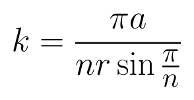## Saturday, November 19, 2011

### Rolling Regular Polygons around a Circle - (1) Polygonal Epicycloid

(n = 5, k = 3)

(n = 3, k = 5/2)

(n = 6, k = 3/2)

(n = 4, k = 1/2)n = the number of sides of the rolling polygon
a = the radius of the base circle
r = the circumradius of the polygon

(n = 3, k = 12/5)

( Mathematical software used: GeoGebra )

Related posts: